### Transition from axi- to nonaxisymmetric dynamo modes in spherical convection models of solar-like stars:

The associated paper can be found here https://arxiv.org/abs/1803.05898.

Visualization of the magnetic field from a rotating simulation.

### Effects of a subadiabatic layer on convection and dynamos in spherical wedge simulations:

The associated paper can be found here https://arxiv.org/abs/1803.05898.

Visualization of the flow from a rotating simulation MHD2 with Ω = 3Ω. The streamlines are colour-coded by the magnitude of the radial velocity Ur. The colour contours at the periphery and on the planes at different depths show the vertical velocity.

Same as above but for a non-rotating simulation HD2.

Visualization of the magnetic field from a rotating simulation MHD2 with Ω = 3Ω. The streamlines are colour-coded by the magnitude of the azimuthal magnetic field Bφ. The colour contours at the periphery and on the planes at different depths show the azimuthal magnetic field.

### Extended subadiabatic layer in overshooting convection:

Vertical velocity Uz and magnetic field Bz near the surface (z = 0.98d), resolution 11523 grid points: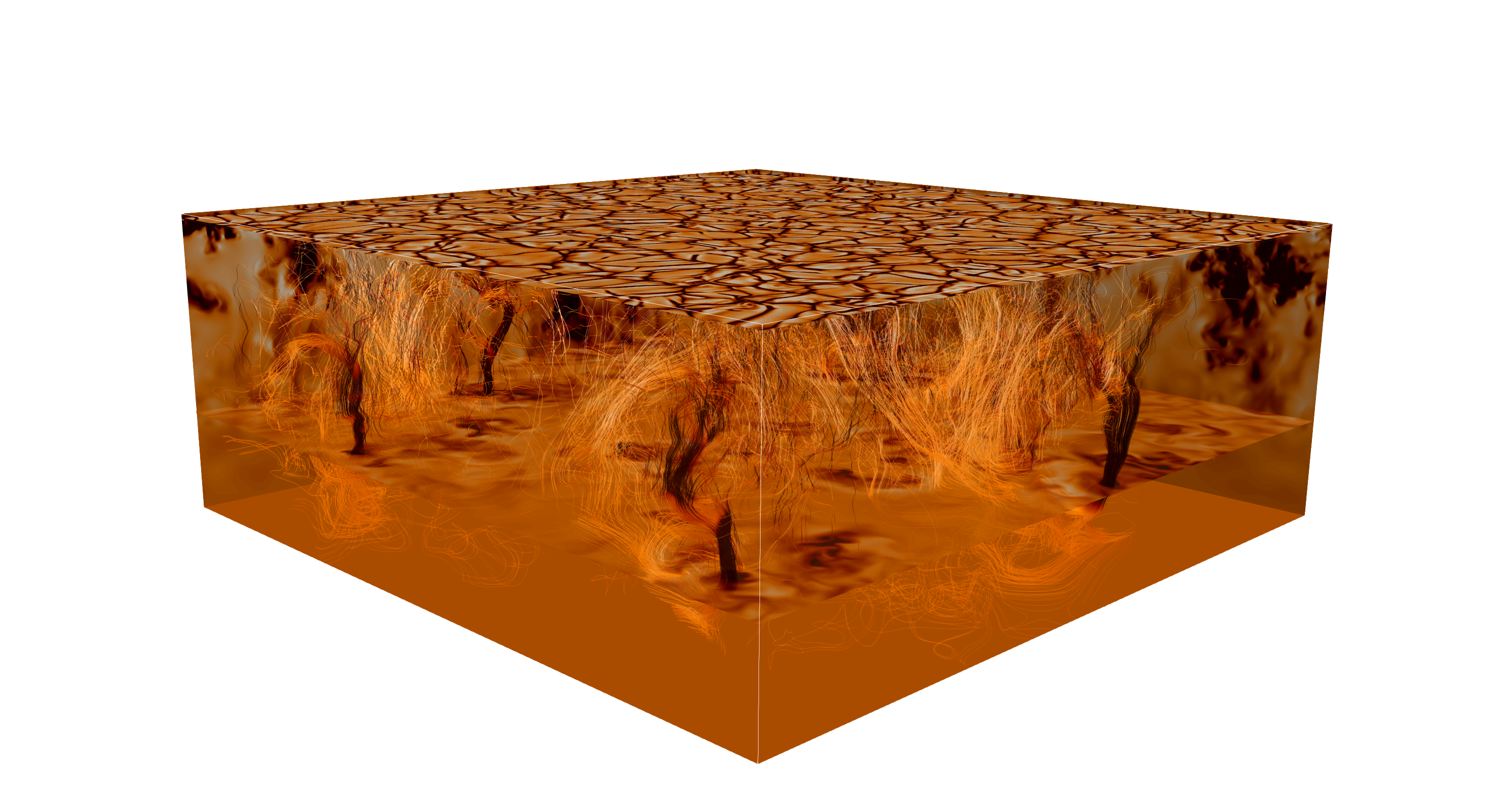### Magnetic flux concentrations from turbulent stratified convection:

Vertical velocity Uz and magnetic field Bz near the surface (z = 0.98d), resolution 10243 grid points: (mp4, 561MB).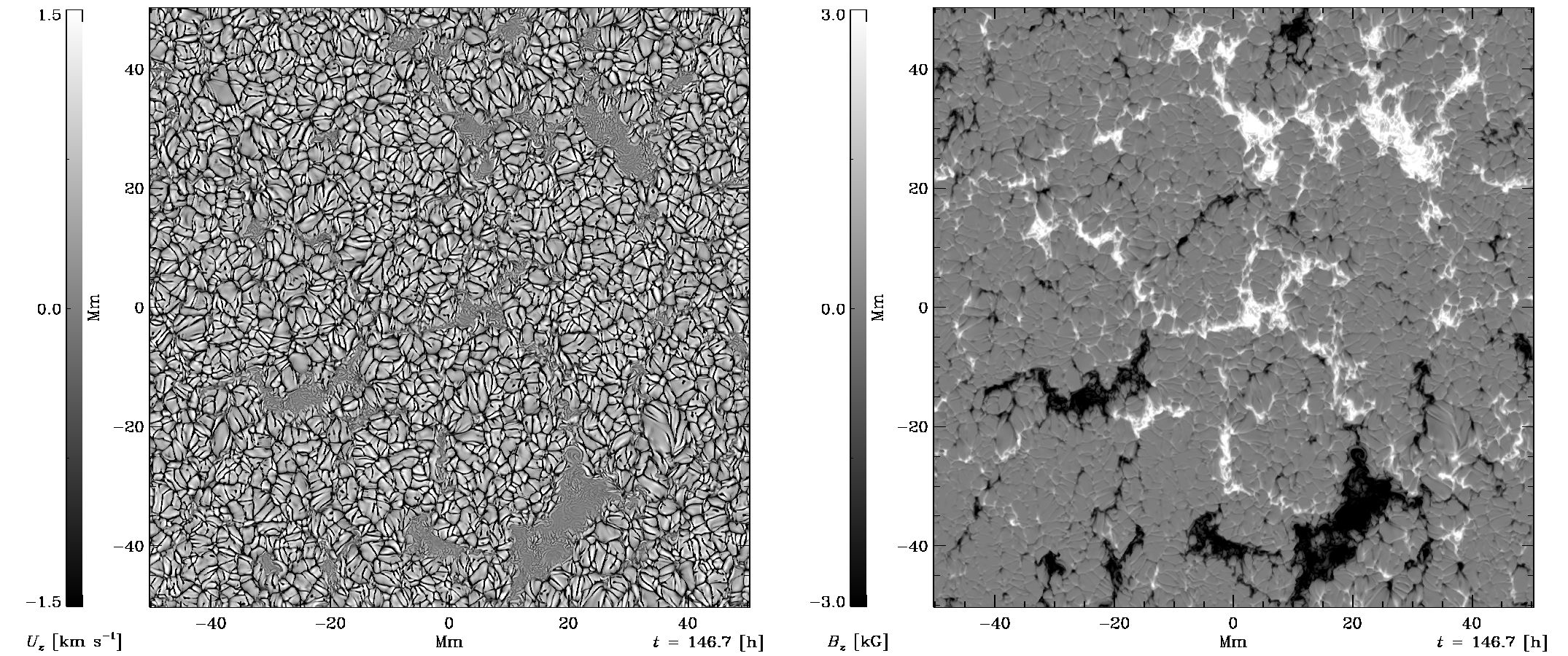### Non-axisymmetric magnetic fields in a convective dynamo simulation:

Longitudinal magnetic field Bφ near the surface (r = 0.98R), Rm ≈ 28 (128 × 256 × 512 grid points): (AVI, 30MB).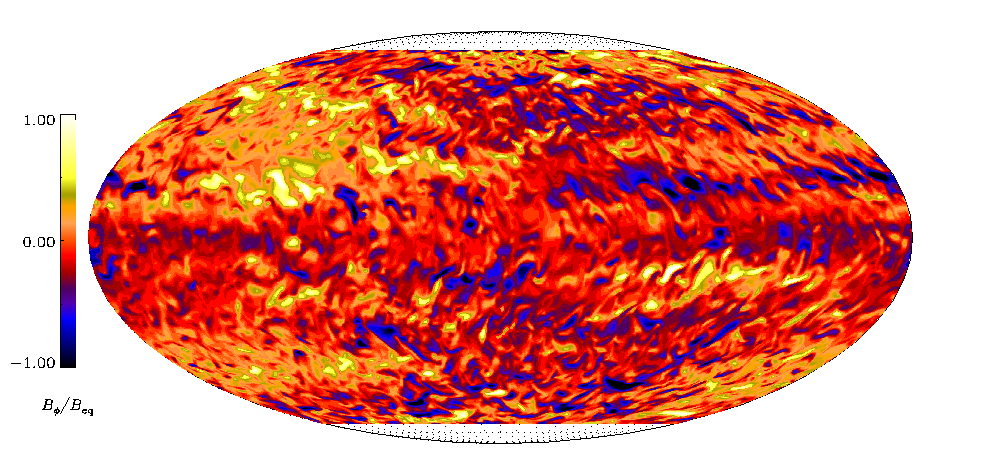### Stratified convection with imposed horizontal magnetic field:

Vertical velocity Uz near the surface (z = 0.98d), Re ≈ 100 (1024 × 512 × 256 grid points): (AVI, 11 MB).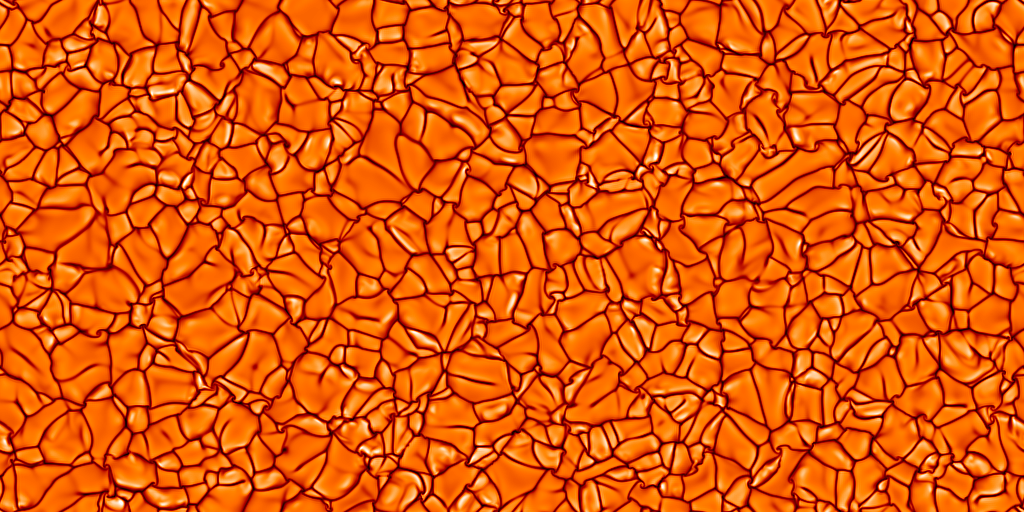Magnetic field component By near the surface (z = 0.98d), Rm ≈ 5 (1024 × 512 × 256 grid points): (AVI, 11 MB).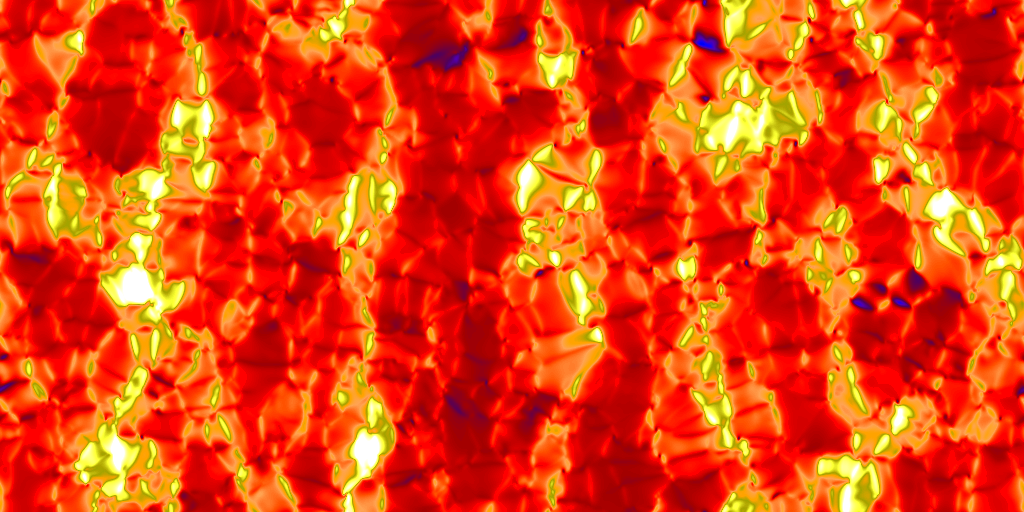Magnetic field component By near the surface (z = 0.98d), Rm ≈ 5 (1024 × 512 × 256 grid points): (AVI, 15 MB).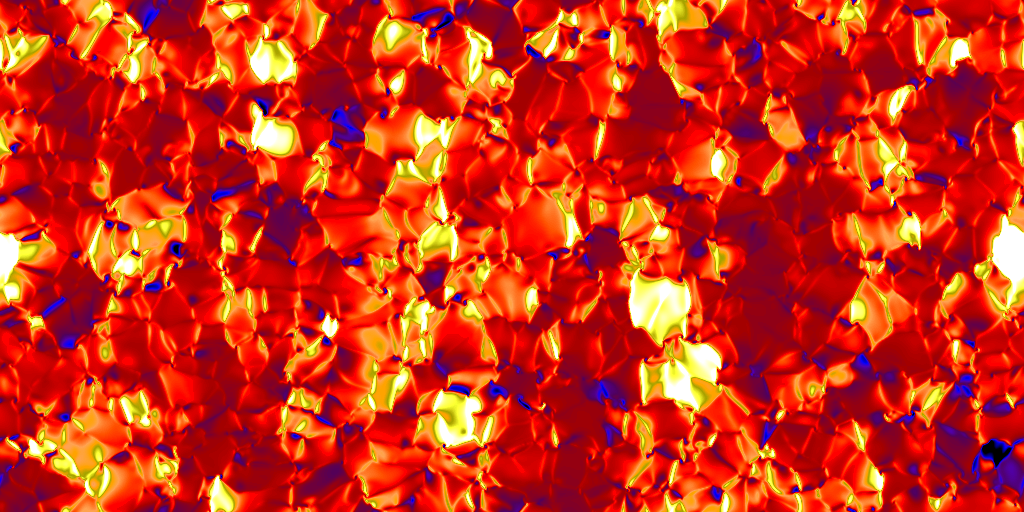Magnetic field component By averaged over the y coordinate, Rm ≈ 5 (1024 × 512 × 256 grid points): (AVI, 2 MB).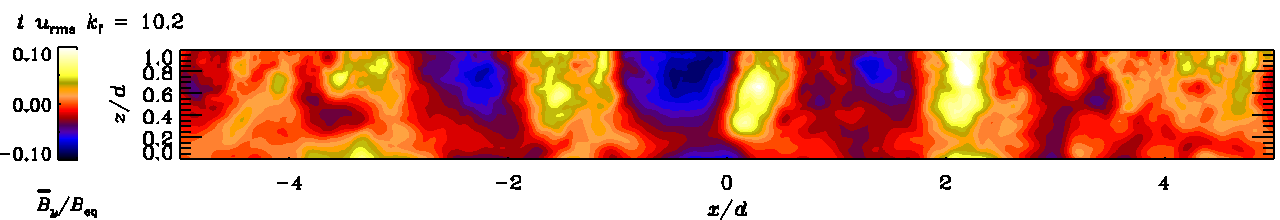Magnetic field component By averaged over the y coordinate, Rm ≈ 20 (1024 × 512 × 256 grid points): (AVI, 2 MB).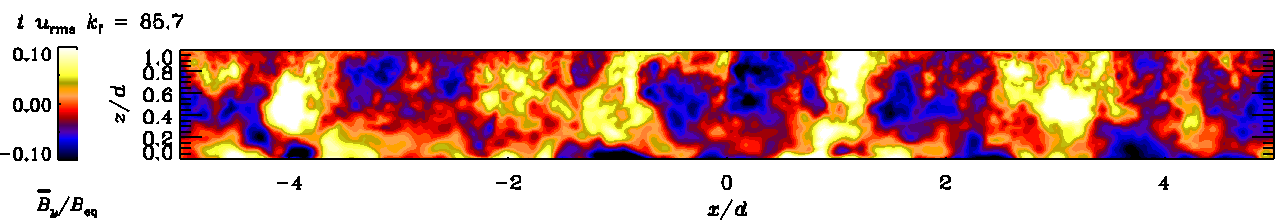### Cyclic magnetic activity due to turbulent convection in spherical wedge geometry:

Longitudinal magnetic field Bφ near the surface (r = 0.98R): (AVI, 68MB).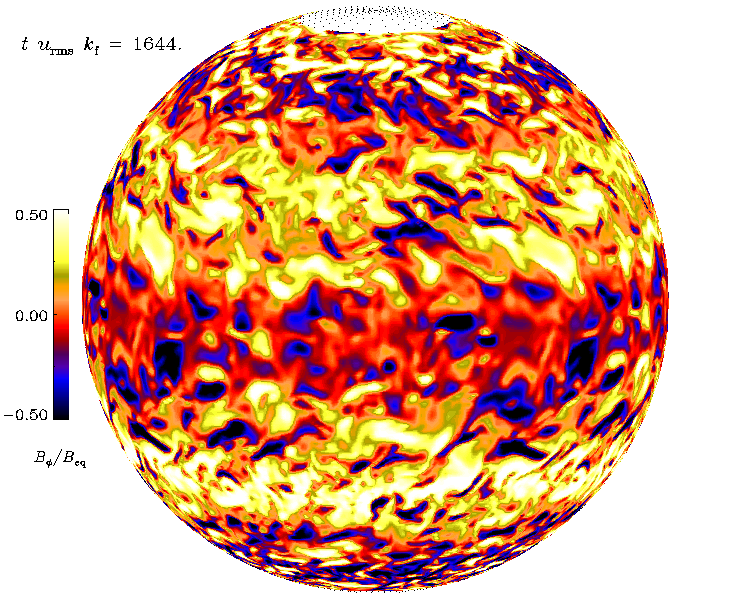### Effects of stratification in spherical shell convection:

Vertical velocity Ur near the surface in 20 simulations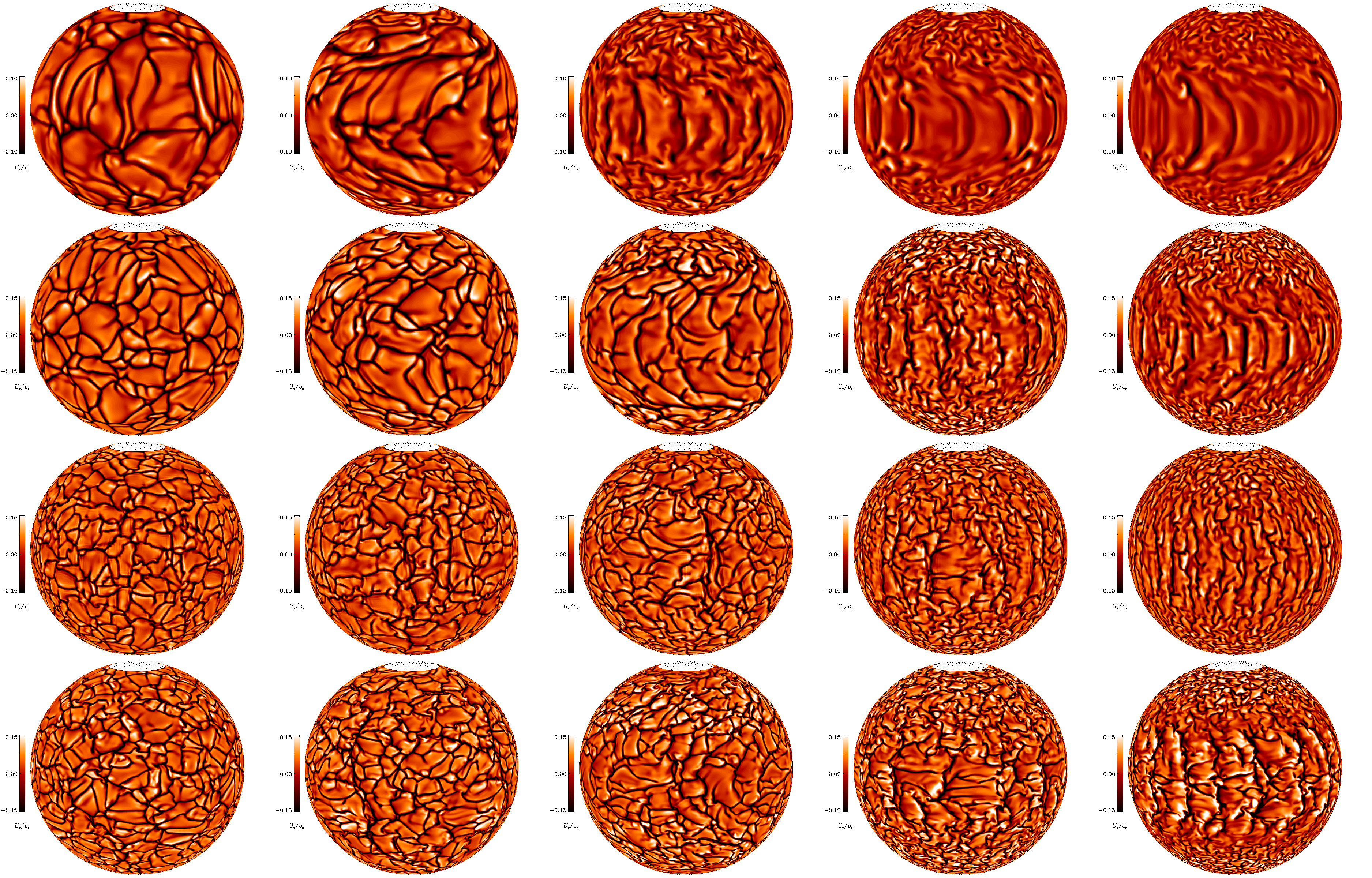### Large-scale cyclones in rotating turbulent convection:

Vertical velocity Uz in the prephery of the domain: (AVI, 15MB), Re ≈ 101, Co ≈ 7.5 (5122 × 256 grid points).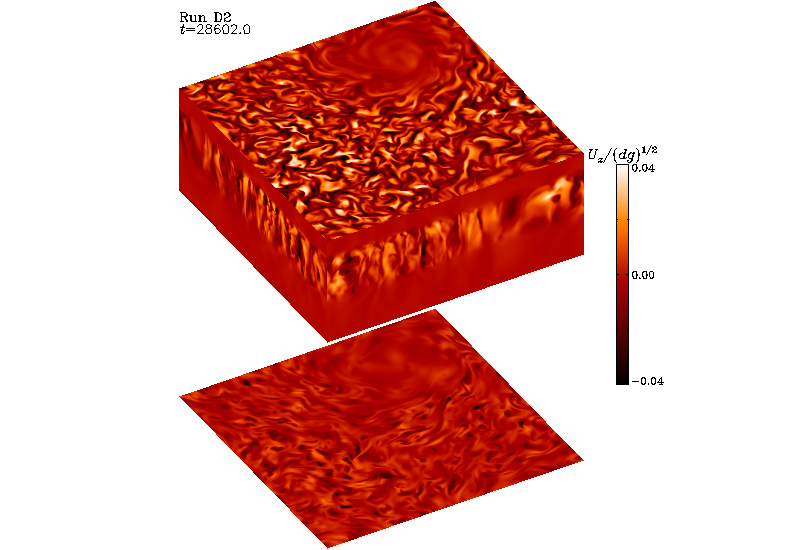### Reynolds stress and heat transport in spherical shell convection:

Snapshots of Ur near the surface from two runs: ±75 degrees latitude, 90 degrees longitude (here duplicated four-fold).

Intermediate rotation with anti-solar differential rotation, Ur: (AVI, 8MB), Re ≈ 57, Co ≈ 1.3 (128 × 512 × 256 grid points).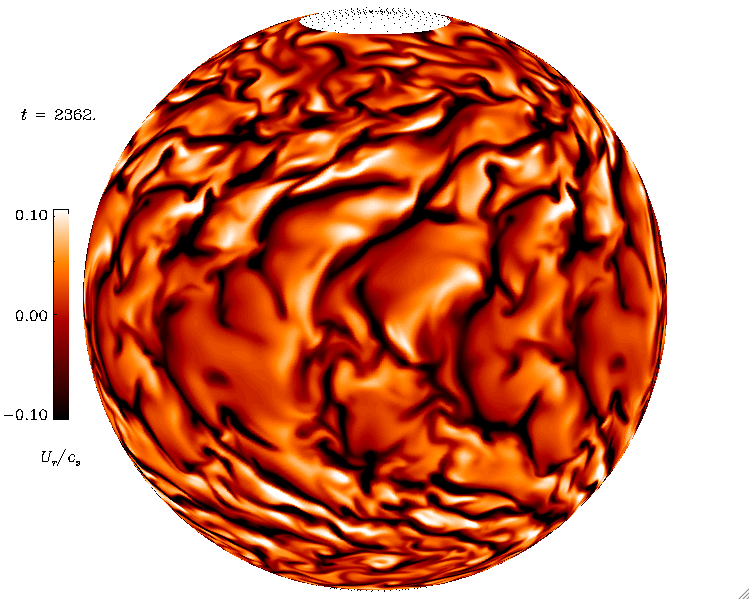Rapid rotation with solar like differential rotation, Ur: (AVI, 5MB), Re ≈ 44, Co ≈ 6.9 (128 × 512 × 256 grid points).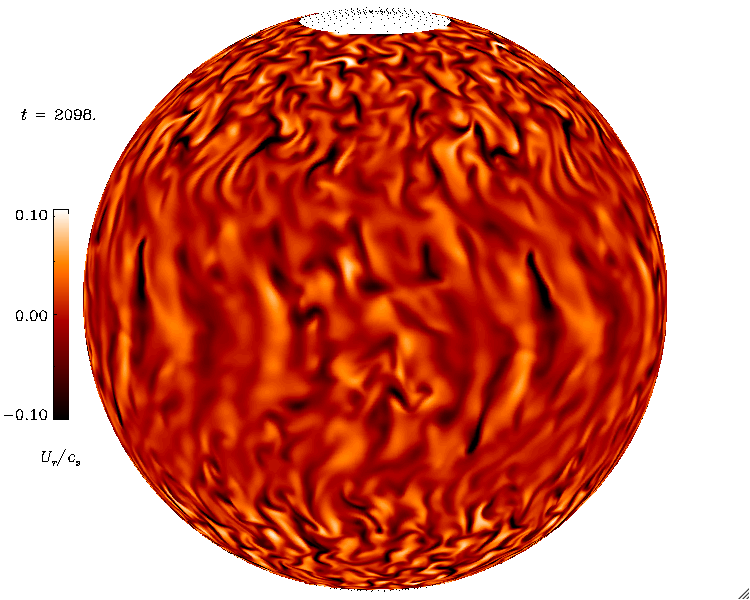### Convection with imposed field:

Imposed vertical field, Bz: (AVI, 8MB), Re ≈ 45, Pm = 1 (2562 × 192 grid points).

Imposed horizontal field, Bx: (AVI, 6MB), Re ≈ 45, Pm = 1 (2562 × 192 grid points).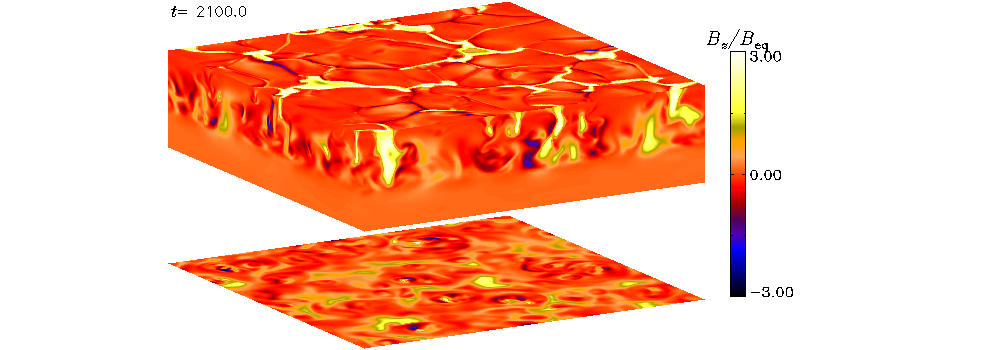### Magnetorotational instability driven dynamos at low magnetic Prandtl numbers:

Velocity component Ux: (AVI, 25MB), Re ≈ 8970, Pm = 0.1 (5123 grid points).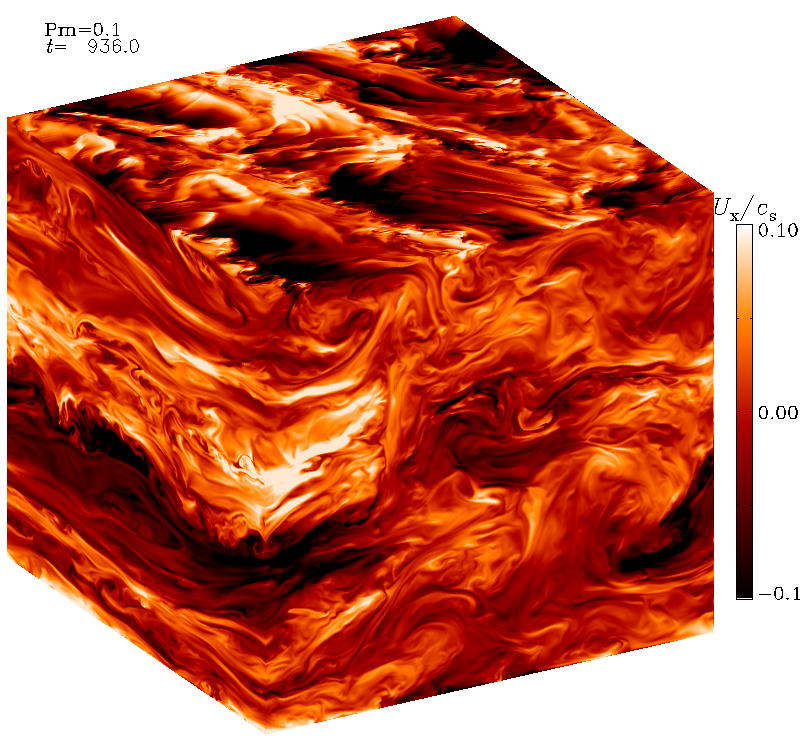### Angular momentum transport in convectively unstable shear flows:

Vertical velocity: (Quicktime, 30MB), Re ≈ 648 (10243 grid points)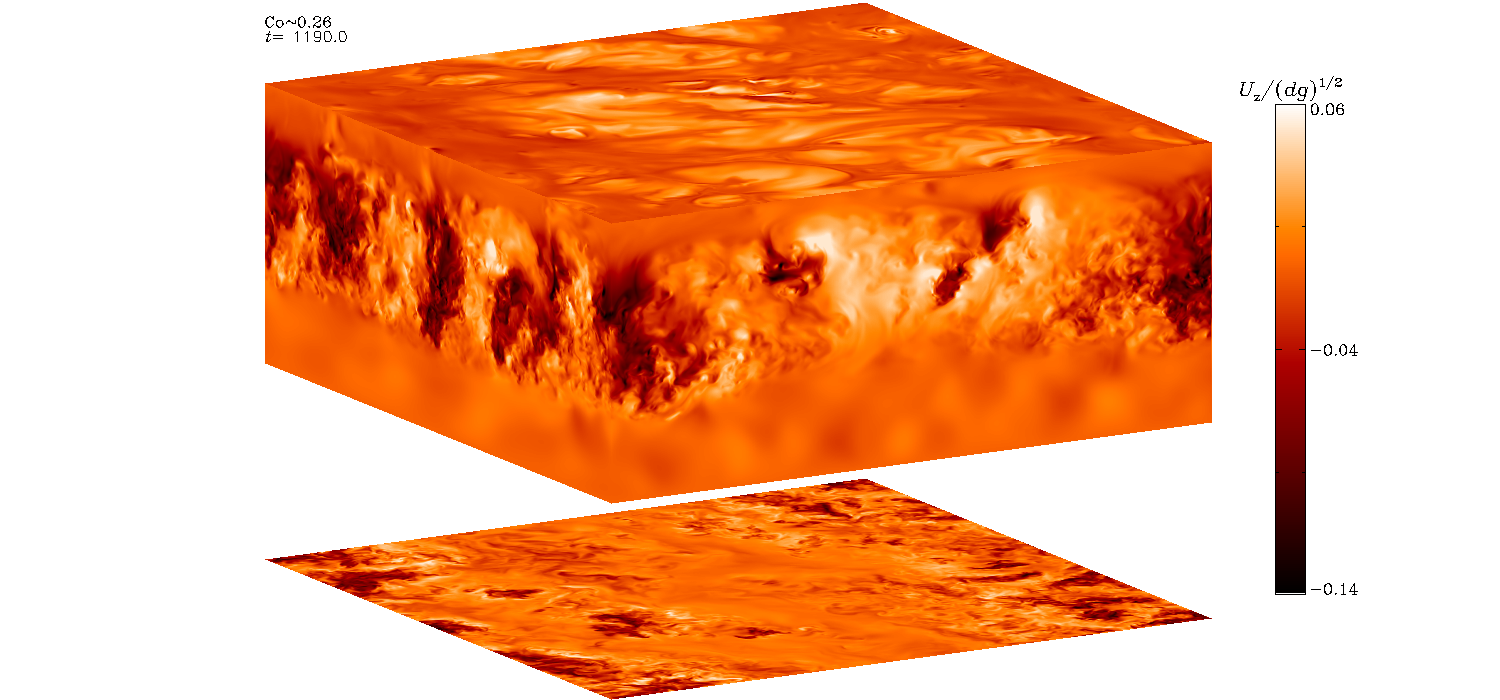### Convective dynamos in spherical wedge geometry:

Vertical velocity Ur near the surface: (Quicktime, 40MB), (DivX, 3MB), Rm ≈ 45.

Azimuthal magnetic field component Bφ near the surface: (Quicktime, 28MB), (DivX, 3MB), Rm ≈ 45.

Azimuthally averaged magnetic field component Bφ: (Quicktime, 20MB), Rm ≈ 30.

### Large-scale dynamos in rigidly rotating turbulent convection:

Magnetic field component Bx: Rm ≈ 90 (DivX 18MB) (5122 × 256 grid points).

Vertical velocity Uz: Re ≈ 45 (DivX 16MB) (5122 × 256 grid points).

### Large-scale dynamos in turbulent convection with shear:

Large-scale magnetic fields in turbulent convection with shear: Rm ≈ 74 (DivX 26MB) (2563 grid points), Rm ≈ 121 (DivX 16MB) (5123 grid points).

Vertical magnetic field at the top of the convectively unstable layer in shearing convection DivX (35MB)